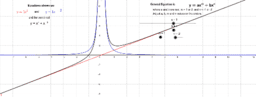Peter22

•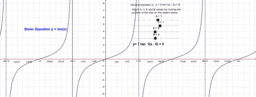Tan Graph with transformations

Activity

Peter22

•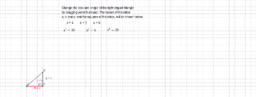Basic Pythagoras

Activity

Peter22

•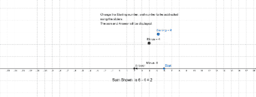Subtraction with positive and negative numbers

Activity

Peter22

•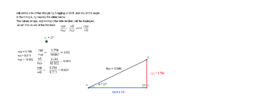Basic Right Angled Triangle Trig ratios

Activity

Peter22

•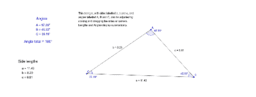Basic Triangle with adjustable side lengths and angles

Activity

Peter22

•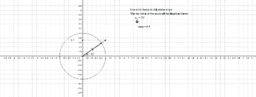Basic Tan 0 to 360 degrees

Activity

Peter22

•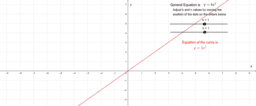y=k x to the power of n

Activity

Peter22

•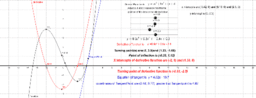Derivative , and Tangent line, for a cubic

Activity

Peter22

•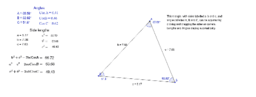Methods collection

Book

Peter22

•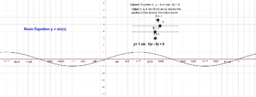Sine Graph with transformations

Activity

Peter22

•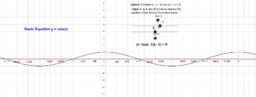Cosine Graph with transformations

Activity

Peter22

•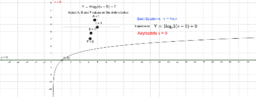Log_e Graph with transformations

Activity

Peter22

•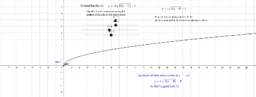Square root graph

Activity

Peter22

•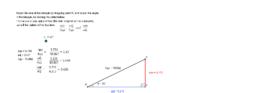Basic Trigonometry with a Right Angled Triangle

Activity

Peter22

•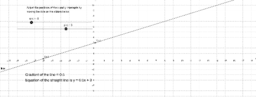Sketch straight line using x and y intercepts

Activity

Peter22

•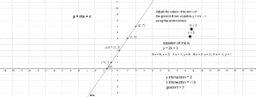Sketching a basic straight line

Activity

Peter22

•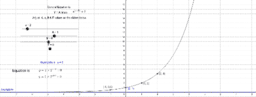Power Exponential Graph

Activity

Peter22

•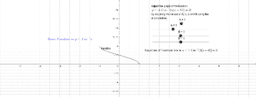Inverse cosine graph

Activity

Peter22

•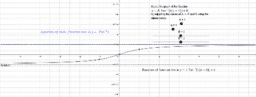Inverse tan graphs

Activity

Peter22

•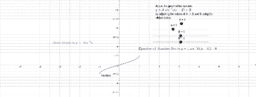Inverse sine Graph and transformations

Activity

Peter22

•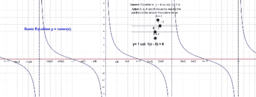Cotan Graph with transformations

Activity

Peter22

•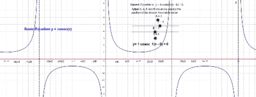Cosec Graph with transformations

Activity

Peter22

•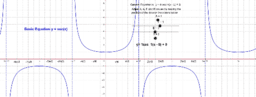Secant Graph with transformations

Activity

Peter22

•